# Polymorphism In Labview

Rating: 4.8

5766

## Polymorphism

All LABVIEW arithmetic functions are polymorphic. This means that the inputs to these functions can be different DATA STRUCTURES such as scalar values and arrays. By definition, polymorphism is said to be the ability of a numeric function to adjust to input data of different data structures.
For example, the Add function can be used in the following ways.
1. Scalar + Scalar = Scalar addition.
2. Scalar + Array = The scalar is added to each element of the array.
3. Array + Array = Each element of one array is added to the corresponding element of another array.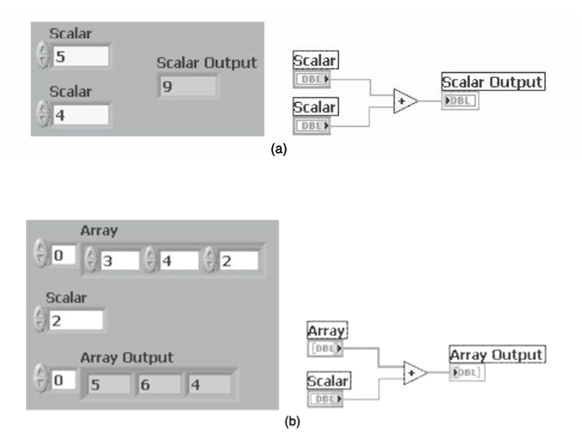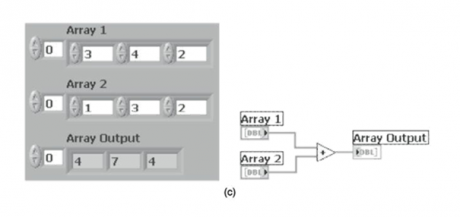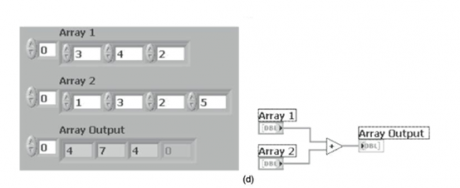In the above Figure (a), two scalar values are added which results in a scalar output. In the above Figure (b), an array is added with a scalar value. The function adds the scalar value to each element of the array and returns the array output. In the above Figure (c), two arrays are added. The function adds each element of one array to the corresponding element of the other array and returns the array output. In the above Figure (d), two arrays of different sizes (i.e. number of factors) are added. The function adds corresponding features and returns the output array, with the size of the smaller input array. The first array consists of three elements and the second consists of four elements. First three elements of both arrays are added. The fourth element of the second array is not considered. Polymorphism does not perform matrix arithmetic when inputs are two-dimensional arrays (for example, two two-dimensional array inputs to a multiply function do element-by-element multiplication, not MATRIX multiplication).## Polymorphism For Numeric Functions

The arithmetic functions take numeric input data. With some exceptions noted in the function descriptions, the default output has the same numeric representation as the input or, if the inputs have different representations, the default output is the larger of the inputs. For example, if you add an 8-bit integer and a 16-bit integer, the default output is a 16-bit integer. If you configure the output of a Numeric function, the settings you specify override the default behavior.

The arithmetic functions work on numbers, arrays of numbers, CLUSTERS of numbers, arrays of clusters of numbers, complex numbers, and so on. A formal and recursive definition of the allowable input type is as follows:
Numeric type = numeric scalar OR array [numeric type] OR cluster [numeric types]

The numeric scalars can be floating-point numbers, integers, or complex floating-point numbers. LABVIEW does not allow you to use arrays of arrays.

Arrays can have any number of dimensions of any size. Clusters can have any number of elements. The output type of functions is of the same numeric representation as the input type. For functions with one input, the functions operate on each element of the array or cluster.

For functions with two inputs, you can use the following input combinations:

• Similar—Both inputs have the same structure, and the output has the same structure as the inputs.
• One scalar—One input is a numeric scalar, the other is an array or cluster, and the output is an array or cluster.
• Array of—One input is a numeric array, the other is the numeric type itself, and the output is an array.

[ Related Article: LabVIEW Tutorials]

For similar inputs, LabVIEW performs the function on the respective elements of the structures. For example, LabVIEW can add two arrays element by element. Both arrays must have the same dimensionality. You can add ARRAYS with differing numbers of elements; the output of such an addition has the same number of elements as the smallest input. Clusters must have the same number of elements, and the respective elements must be of the same type.

For operations involving a scalar and an array or cluster, LabVIEW performs the function on the scalar and the respective elements of the structure. For example, LabVIEW can subtract a number from all elements of an array, regardless of the dimensionality of the array.

For operations that involve a numeric type and an array of that type, LabVIEW performs the function on each array element. For example, a graph is an array of points, and a point is a cluster of two numeric types, x and y. To offset a graph by 5 units in the x direction and 8 units in the y direction, you can add a point, (5, 8), to the graph.

The following illustration shows the possible polymorphic combinations of the Add function.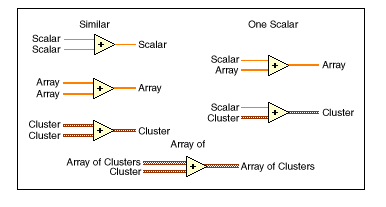[Related Article: Deloitte Interview Questions]

## Polymorphism For Boolean Functions

The logical functions accept Boolean input data, numeric input data, and error clusters. If the input is numeric, LabVIEW performs a bitwise operation. If the input is an integer, the output has the same representation. If the input is a floating-point number, LabVIEW rounds it to a 32-bit integer, and the output is a 32-bit integer. If the input is an error cluster, LabVIEW passes only the TRUE or FALSE value of the status parameter of the error cluster to the input terminal.

The logical functions work on arrays of numbers or Boolean values, clusters of numbers or Boolean values, arrays of clusters of numbers or Boolean values, and so on.
A formal and recursive definition of the allowable input type is as follows:

Logical type = Boolean scalar OR numeric scalar OR array [logical type] OR cluster [logical types]
except that complex numbers and arrays of arrays are not allowed.

Logical functions with two inputs can have the same input combinations as the arithmetic functions. However, the logical functions have the further restriction that the base operations can only be between two Boolean values or two numbers. For example, you cannot have an AND between a Boolean value and a number.

The following illustration shows some combinations of Boolean values for the AND function.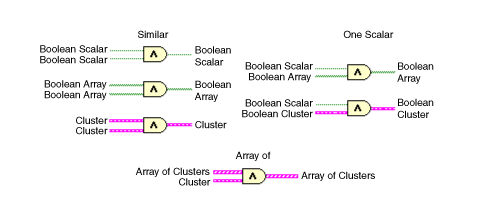Course Schedule
NameDates
LabVIEW TrainingJun 03 to Jun 18
LabVIEW TrainingJun 06 to Jun 21
LabVIEW TrainingJun 10 to Jun 25
LabVIEW TrainingJun 13 to Jun 28
Last updated: 02 June 2023